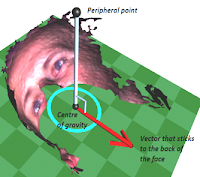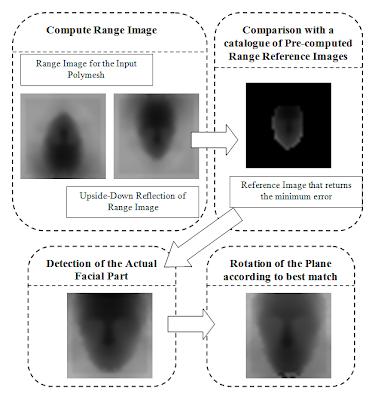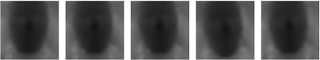## Tuesday, 4 September 2012

### Geometrical Correspondence Tools for Facial Animation Production

The project I most enjoyed is my undergraduate individual project with supervisor Dr Tilo Burghardt from University of Bristol.

## 1. Motivation

A project carried out at Cardiff University captures the motion of a human’s face and produces a sequence of 48 spatio-temporal models per second using 3dMDFaceTM Dynamic System . But those facial scans are really noisy and around 10TB are required for a 10mins video. In order to merge them into a single representation and improve their quality, semantically identical facial elements should be associated between the modes.

## 2. Related Work

1. Accurate registration based on symmetry plane around nose
[X.M Tang, J.S. Chen and Y.S Moon. Accurate 3D face registration based on the symmetry plane analysis of nose region. 2008]
2. Real-time Face Pose Estimation
[Michael D.Breinstein, Daniel Kuettel, Thibaut Weise, Luc Van Goolm Hanspeter Pfister. Real-time Face Pose Estimation from Single Range Images. 
3. Constructing a realistic Face Model of An Individual for Expression Animation
[Yu Zhang, Edmond C. Prakash and Eric Sung. Constructing a  realistic Face Model of An

## 3. Outline of the Application

That project is able to resolve the approximate position of key features from a facial points cloud.

It is robust in terms of:

1.         Scaling
2.         Rotation
3.         Translation
4.         Noise

It is achieved in four stages as shown below:Figure 1: Outline of the Application

## 4. Plane Initialisation

Initialise a plane that is approximately aligned with the face. Find a few peripheral points and used then to find an amount of backwards-pointing vectors. The initial plane passes through the centre of gravity and its normal is the average of those vectors.Figure 2: Calculation of a backwards pointing vector

## 5. Iterative Algorithm

An iterative Algorithm with three degrees of freedom is used to find the bilateral symmetry axis of the face.

The iterative algorithm has 3 sub-iterations; each one serves a degree of freedom:
1.         Rotation of the plane around its normal.
2.         Shifting the plane left and right.
3.         Rotation of the plane around the symmetry axis.

During each iteration the mirror difference is calculated.Figure 3: Mirror Difference of the face, P is an array with sorted points  that lie on the plane that is aligned with the face

During each sub-iteration, the mirror difference (A2, A3 and A4) is calculated for input values B2, B3 and B4. After each sub-iteration, the algorithm divides its observation field and repeats until the observation field become too small to be divided again.Figure 4: How the  observation field is halved during each iteration
The sub-iterations run the one after the other. Once they all converge, the system runs them again and repeats until no changes are observed.

## 6. Posing a Template

By posing a template the plane is rotated in another degree of freedom and the actual facial part is detected.Figure 5: Comparison with pre-computed reference of range images, using an Error Function

## 7. Detection of Key Points:

Once the face is aligned, the approximate position of key Points are detected using curvature analysis and outward projection of points.

## 8. Evaluation:

The following image shows the evaluation of the program:Figure 8:  Initial InvarianceFigure 9: Symmetry axisFigure 10: Range ImagesFigure 11: Key Points

## 9. Future Work

Once the features of interest are found:

1.         Polymesh sequences can be merged into a single representation
2.         Mesh quality can be improved and
3.         Their motion can be driven by another model.

The facial registration system is also a useful tool in expression analysis and face recognition systems.